﻿ 5. Detailed description of the Actions > 5.5. Standard > 5.5.6. Calculator (High-Speed action)

# 5.5.6. Calculator (High-Speed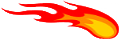action)

Navigation:  5. Detailed description of the Actions > 5.5. Standard >

# 5.5.6. Calculator (High-Speedaction)

Icon: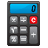Function: Calculator

Property window: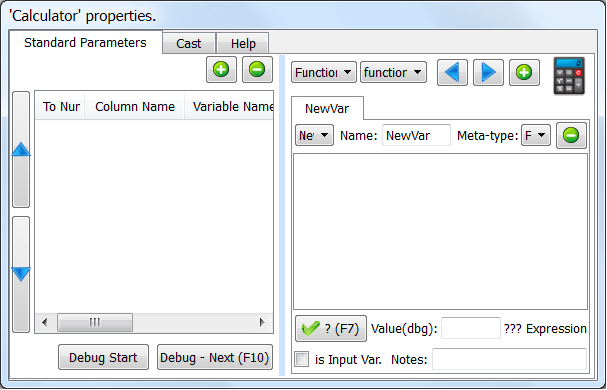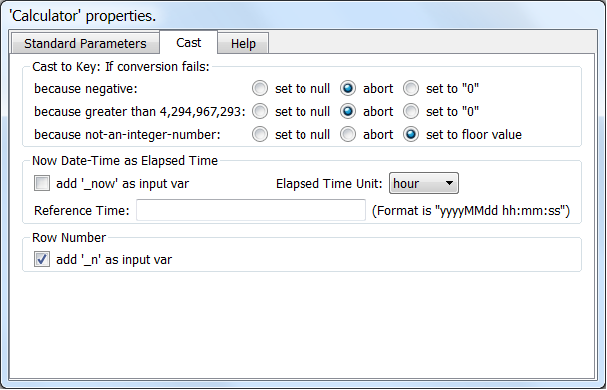Short description:

This creates or updates new columns based on the other columns in the current row.

Long Description:

Here is a first example: We want to compute the “NetProfit” based on the columns “Margin” and “Operating Cost” (NetProfit=Margin-Operating Cost). We’ll have: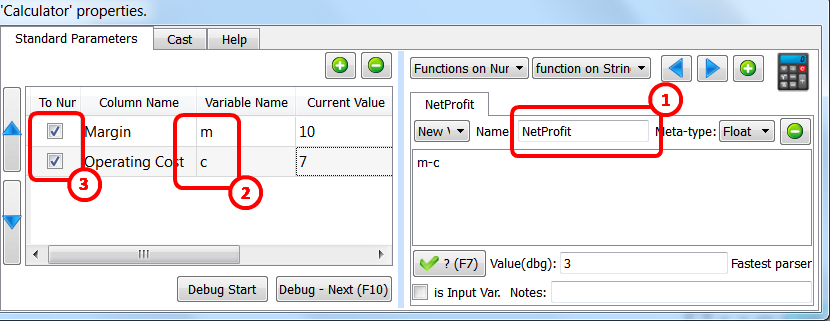Some remarks about the above example:

a)We created a new column named “NetProfit”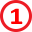b)To compute “NetProfit”, we used two other columns: “Margin” and “Operating Cost”. Inside the mathematical expression defining the “NetProfit” these 2 columns were, respectively, named “m” and “c”: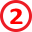“m” and “c” are variables inside the mathematical expression “m-c” defining the “NetProfit”. Variable names can use the following characters: a-z,A-Z,0-9,_ (spaces are not allowed in variables names). Variable names cannot start with a number (thus “1A” is an invalid variable name but “_1A” is ok).

c)Variables (such as “m” and “c”) can either be of the numerical type or of string type.

You define here: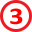the type (number or string) of each of the variables (in the above example, the 2 variables are of the number type, thus the 2 checkboxes are both enabled).

There are no automatic conversions from one type to another: you must use the function atof (to convert a string to a floating-point number), atoi (to convert a string to an integer number), ftoa (to convert a floating-point number to a string) to compute the conversions.

Most operators require a specific type to work: For example, you cannot multiply together two variables of the string type: only variables of the numerical type can be multiplied together.

d)You have a description of all the available operators inside the “Help” tab of theCalculator Action: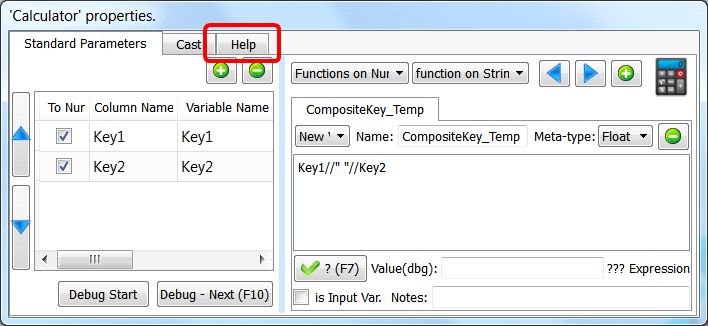e)To check if your mathematical expression is valid (e.g. to check if you used the required variable type: numerical or string), you can click the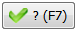button:Anatella will use the “Current Value”of each variable to evaluate your mathematical expression and, if there are no errors, it will give the result here::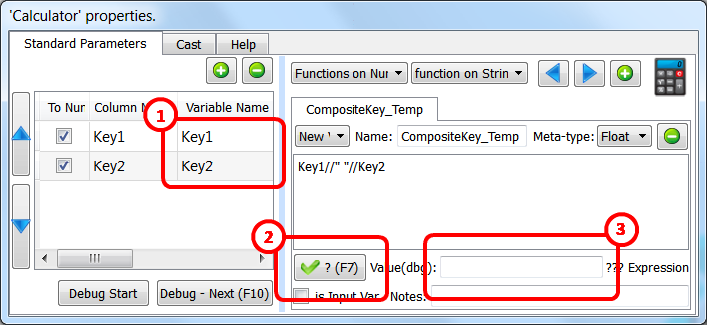When you click thebutton, you get: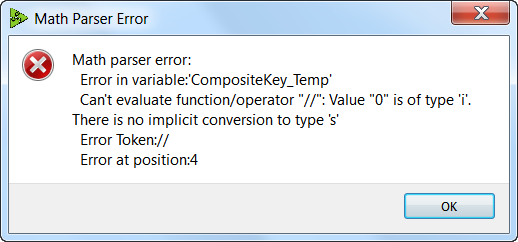The above error message tells you that your variable types are wrong: You defined your 2 variables “Key1” and “Key2” as “integers” (i.e. type ‘i’) but you used them with the string-concatenation-operator (the “//”) that requires the type ‘s’ (i.e. the string type). The solution is simple: click here: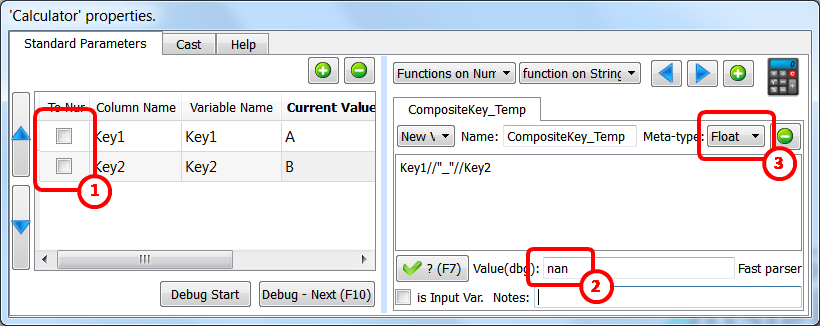When you click thebutton again, you get no errors but the result is strange:(We were expecting “A_B”, as result, but we got “nan” instead). How did we obtain “nan”? The answer is simple: Anatella evaluates your expression and correctly obtains “A_B” but, after that, it attempts to store the result “A_B” into a column of the floating-point type:The result of the conversion of “A_B” to a floating-point number is “nan” (i.e. “Not-A-Number). To correct this, change the meta-type of the new column: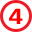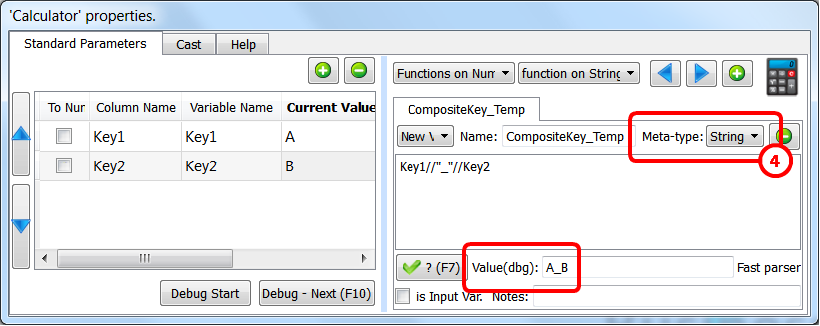f)Once Anatella has finished evaluating your mathematical expression, it stores the result into the (new) column inside the output table of theCalculator Action. The Meta-type of the (new) column must be compatible with the result’s type (otherwise you get strange results such as the “nan” result of the example on the previous page). In particular, the conversion of the result to the “Key” Meta-type might fail for different reasons. Use these settings: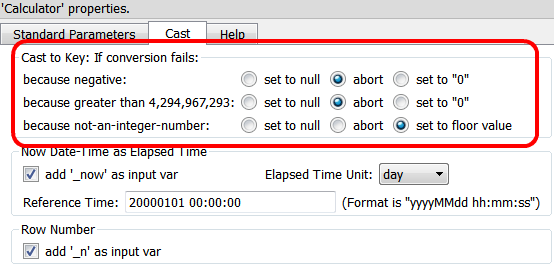to parameterize how Anatella handles the conversion to the “Key” Meta-type: